# Parallel Connection of Coupled Coils

## Parallel Connection of Coupled Coils:

Parallel Connection of Coupled Coils – Consider two inductors with self inductances L1 and L2 connected parallel which are mutually coupled with mutual inductance M as shown in Fig. 10.20.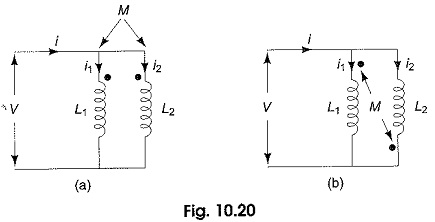Let us consider Fig. 10.20(a) where the self induced emf n each coil assists the mutually induced emf as shown by the dot convention.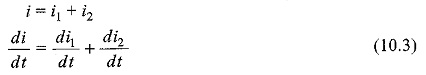The voltage across the parallel branch is given by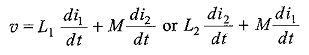also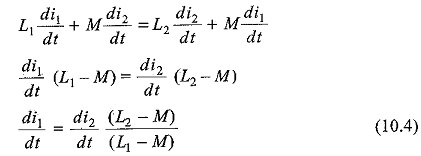Substituting Eq. 10.4 in Eq. 10.3, we get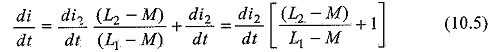If Leq is the equivalent inductance of the Parallel Connection of Coupled Coils in Fig 10.20 (a) then υ is given by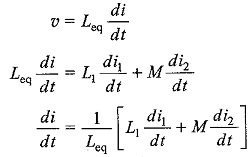Substituting Eq. 10.4 in the above equation we get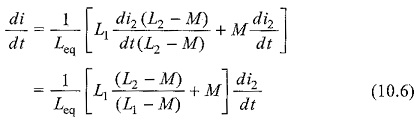Equating Eq. 10.6 and Eq. 10.5, we get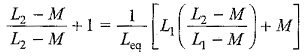Rearranging and simplifying the above equation results in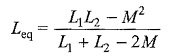If the voltage induced due to mutual inductance oppose the self induced emf in each coil as shown by the dot convention in Fig. 10.20(b), the equivalent inductance is given by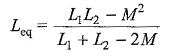Updated: December 28, 2019 — 1:26 pm